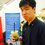# Is there a better way to solve this problem than using the Sophie-Germain Identity?

http://www.artofproblemsolving.com/Wiki/index.php/1987AIMEProblems/Problem_14

Evaluate $$\frac{(10^4+324)(22^4+324)(34^4+324)(46^4+324)(58^4+324)}{(4^4+324)(16^4+324)(28^4+324)(40^4+324)(52^4+324)}$$.

I'd be surprised if it was because usually MAA doesn't require you to know relatively obscure theorems in their competitions until you get to at least USAMO. Is there a more intuitive way to solve this problem?

CheersNote by Michael Tong
7 years, 1 month ago

This discussion board is a place to discuss our Daily Challenges and the math and science related to those challenges. Explanations are more than just a solution — they should explain the steps and thinking strategies that you used to obtain the solution. Comments should further the discussion of math and science.

When posting on Brilliant:

• Use the emojis to react to an explanation, whether you're congratulating a job well done , or just really confused .
• Ask specific questions about the challenge or the steps in somebody's explanation. Well-posed questions can add a lot to the discussion, but posting "I don't understand!" doesn't help anyone.
• Try to contribute something new to the discussion, whether it is an extension, generalization or other idea related to the challenge.

MarkdownAppears as
*italics* or _italics_ italics
**bold** or __bold__ bold
- bulleted- list
• bulleted
• list
1. numbered2. list
1. numbered
2. list
Note: you must add a full line of space before and after lists for them to show up correctly
paragraph 1paragraph 2

paragraph 1

paragraph 2

[example link](https://brilliant.org)example link
> This is a quote
This is a quote
    # I indented these lines
# 4 spaces, and now they show
# up as a code block.

print "hello world"
# I indented these lines
# 4 spaces, and now they show
# up as a code block.

print "hello world"
MathAppears as
Remember to wrap math in $$ ... $$ or $ ... $ to ensure proper formatting.
2 \times 3 $2 \times 3$
2^{34} $2^{34}$
a_{i-1} $a_{i-1}$
\frac{2}{3} $\frac{2}{3}$
\sqrt{2} $\sqrt{2}$
\sum_{i=1}^3 $\sum_{i=1}^3$
\sin \theta $\sin \theta$
\boxed{123} $\boxed{123}$

Sort by:

Actually, sophie-germain identity isn't very obscure. Well.. not as well known as difference of squares of difference of cubes though...

- 7 years, 1 month ago

Seems like once I got this problem in Brilliant too...

- 7 years, 1 month ago

Yes me too

- 7 years ago

Notice that all of the fourth powers differ be $12$. Try using a substitution that takes advantage of this and see if you can somehow simplify the result.

- 7 years ago

What I meant was, the fourth powers in the numerator all differ by $12$ and all the fourth powers in the denominator differ by $12$.

- 7 years ago

See this.

- 4 years, 12 months ago